# Sum of series

Determine the 6-th member and the sum of a geometric series:

5-4/1+16/5-64/25+256/125-1024/625+....

Result

a6 =  -1.64
SUM =  2.78

#### Solution:Leave us a comment of example and its solution (i.e. if it is still somewhat unclear...):Be the first to comment!#### To solve this verbal math problem are needed these knowledge from mathematics:

Need help calculate sum, simplify or multiply fractions? Try our fraction calculator.

## Next similar examples:

1. Infinite decimalImagine the infinite decimal number 0.99999999 .. ... ... ... That is a decimal and her endless serie of nines. Determine how much this number is less than the number 1. Thank you in advance.
2. Series and sequencesFind a fraction equivalent to the recurring decimal? 0.435643564356
3. Hot air balloon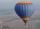Hot air balloon ascends 25 meters up for a minute after launch. Every minute ascends 75 percent of the height which climbed in the previous minute. a) how many meters ascends six minutes after takeoff? b) what is the overall height 10 minutes after launch
4. Five membersWrite first 5 members geometric sequence and determine whether it is increasing or decreasing: a1 = 3 q = -2
5. GP - 8 itemsDetermine the first eight members of a geometric progression if a9=512, q=2
6. Tenth memberCalculate the tenth member of geometric sequence when given: a1=1/2 and q=2
7. Geometric progression 2There is geometric sequence with a1=5.7 and quotient q=-2.5. Calculate a17.
8. Six termsFind the first six terms of the sequence a1 = -3, an = 2 * an-1
9. Geometric progression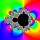Fill 4 numbers between 4 and -12500 to form geometric progression.
10. Piano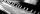If Suzan practicing 10 minutes at Monday; every other day she wants to practice 2 times as much as the previous day, how many hours and minutes will have to practice on Friday?
11. Ray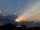Light ray loses 1/19 of brightness passing through glass plate. What is the brightness of the ray after passing through 7 identical plates?
12. Classroom 4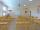In a class of 36 pupils, 2/3 are girls. How much it is in a class girls and boys?
13. Theorem proveWe want to prove the sentence: If the natural number n is divisible by six, then n is divisible by three. From what assumption we started?
14. Icecreams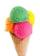Karthik bought 30 icecreams for his birthday .2/5 of them are chocolate icecreams. Find the number of chocolate icecreams he bought?
15. Watching TVOne evening 2/3 students watch TV. Of those students, 3/8 watched a reality show. Of the students that watched the show, 1/4 of them recorded it. What fraction of the students watched and recorded reality tv.
16. Pie II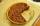Vili ate three pieces of pie. If it pieces is 1/8 how much pie did he eat?
17. Write 3Write a real world problem involving the multiplication of a fraction and a whole number with a product that is between 8 and 10 then solve the problem